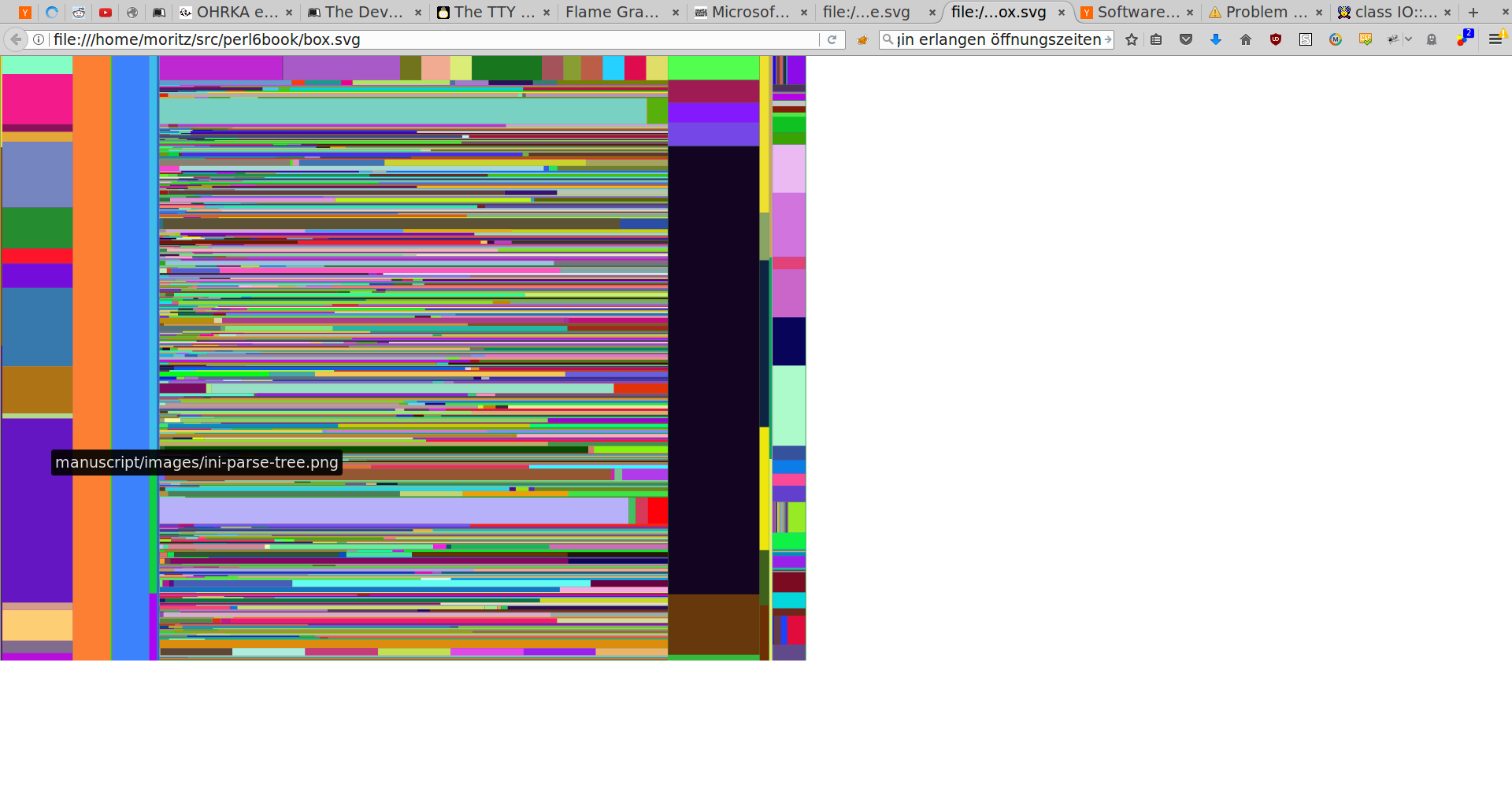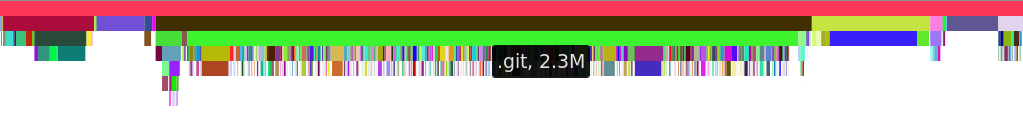# Perl 6 By Example: A File and Directory Usage GraphThis blog post is part of my ongoing project to write a book about Perl 6.

If you're interested, either in this book project or any other Perl 6 book news, please sign up for the mailing list at the bottom of the article, or here. It will be low volume (less than an email per month, on average).

# A File and Directory Usage Graph

You bought a shiny new 2TB disk just a short while ago, and you're already getting low disk space warnings. What's taking up all that space?

To answer this question, and experiment a bit with data visualization, let's write a small tool that visualizes which files use up how much disk space.

To do that, we must first recursively read all directories and files in a given directory, and record their sizes. To get a listing of all elements in a directory, we can use the dir function, which returns a lazy list of IO::Path objects.

We distinguish between directories, which can have child entries, and files, which don't. Both can have a direct size, and in the case of directories also a total size, which includes files and subdirectories, recursively:

``````class File {
has \$.name;
has \$.size;
method total-size() { \$.size }
}

class Directory {
has \$.name;
has \$.size;
has @.children;
has \$!total-size;
method total-size() {
\$!total-size //= \$.size + @.children.map({.total-size}).sum;
}
}

sub tree(IO::Path \$path) {
if \$path.d {
return Directory.new(
name     => \$path.basename,
size     => \$path.s,
children => dir(\$path).map(&tree),
);
}
else {
return File.new(
name => \$path.Str,
size => \$path.s,
);
}
}
``````

Method `total-size` in class `Directory` uses the construct ```\$var //= EXPR´. The```//```stands for *defined-OR*, so it returns the left-hand side if it has a defined value. Otherwise, it evalutes and returns the value of```EXPR`. Combined with the assignment operator, it evaluates the right-hand side only if the variable is undefined, and then stores the value of the expression in the variable. That's a short way to write a cache.

The code for reading a file tree recursively uses the `d` and `s` methods on `IO::Path`. `d` returns True for directories, and false for files. `s` returns the size. (Note that `.s` on directories used to throw an exception in older Rakudo versions. You must use Rakudo 2017.01-169 or newer for this to work; if you are stuck on an older version of Rakudo, you could hard code the size of a directory to a typical block size, like 4096 bytes. It typically won't skew your results too much).

Just to check that we've got a sensible data structure, we can write a short routine that prints it recursively, with indention to indicate nesting of directory entries:

``````sub print-tree(\$tree, Int \$indent = 0) {
say ' ' x \$indent, format-size(\$tree.total-size), '  ', \$tree.name;
if \$tree ~~ Directory {
print-tree(\$_, \$indent + 2) for \$tree.children
}
}

sub format-size(Int \$bytes) {
my @units = flat '', <k M G T P>;
my @steps = (1, { \$_ * 1024 } ... *).head(6);
for @steps.kv -> \$idx, \$step {
my \$in-unit = \$bytes / \$step;
if \$in-unit < 1024 {
return sprintf '%.1f%s', \$in-unit, @units[\$idx];
}
}
}

sub MAIN(\$dir = '.') {
print-tree(tree(\$dir.IO));
}
``````

The subroutine `print-tree` is pretty boring, if you're used to recursion. It prins out the name and size of the current node, and if the current node is a directory, recurses into each children with an increased indention. The indention is applied through the `x` string repetition operator, which when called as `\$string x \$count` repeates the `\$string` for `\$count` times.

To get a human-readable repretation of the size of a number, `format-size` knows a list of six units: the empty string for one, `k` (kilo) for 1024, `M` (mega) for 1024*1024 and so on. This list is stored in the array `@units`. The multiplies assoziated with each unit is stored in `@steps`, which is iniitliazed through the series operator. `...`. Its structure is `INITIAL, CALLABLE ... LIMIT`, and repeatedly applies `CALLABLE` first to the initial value, and then to next value generated and so on, until it hits `LIMIT`. The limit here is `*`, a special term called Whatever, which in this means it's unlimited. So the sequence operator returns a lazy, potentially infinite list, and the tailing `.head(6)` call limits it to 6 values.

To find the most appropriate unit to print the size in, we have to iterate over both the values and in the indexes of the array, which ```for @steps.kv -> \$idx, \$step { .. }``` accomplishes. `sprintf`, known from other programming languages, does the actual formatting to one digit after the dot, and appends the unit.

## Generating a Tree Map

One possible visualization of file and directory sizes is a tree map, which represents each directory as a rectangle, and a each file inside it as a rectangle inside directory's rectangle. The size of each rectangle is proportional to the size of the file or directory it represents.

We'll generate an SVG file containing all those rectangles. Modern browsers support displaying those files, and also show mouse-over texts for each rectangle. This alleviates the burden to actually label the rectangnles, which can be quite a hassle.

To generate the SVG, we'll use the `SVG` module, which you can install with

``````\$ zef install SVG
``````

or

``````\$ panda install SVG
``````

depending on the module installer you have available.

This module provides a single static method, to which you pass nested pairs. Pairs whose values are arrays are turned into XML tags, and other pairs are turned into attributes. For example this Perl 6 script

``````use SVG;
print SVG.serialize(
:svg[
width => 100,
height => 20,
title => [
'example',
]
],
);
``````

produces this output:

``````<svg xmlns="http://www.w3.org/2000/svg"
xmlns:svg="http://www.w3.org/2000/svg"
width="100"
height="20">
<title>example</title>
</svg>
``````

(without the indention). The `xmlns`-tags are helpfully added by the `SVG` module, and are necessary for programs to recognize the file as SVG.

To return to the tree maps, a very simple way to lay out the rectangle is to recurse into areas, and for each area subdivide it either horizontally or vertically, depending on which axis is longer:

``````sub tree-map(\$tree, :\$x1!, :\$x2!, :\$y1!, :\$y2) {
# do not produce rectangles for small files/dirs
return if (\$x2 - \$x1) * (\$y2 - \$y1) < 20;

# produce a rectangle for the current file or dir
take 'rect' => [
x      => \$x1,
y      => \$y1,
width  => \$x2 - \$x1,
height => \$y2 - \$y1,
style  => "fill:" ~ random-color(),
title  => [\$tree.name],
];
return if \$tree ~~ File;

if \$x2 - \$x1 > \$y2 - \$y1 {
# split along the x axis
my \$base = (\$x2 - \$x1) / \$tree.total-size;
my \$new-x = \$x1;
for \$tree.children -> \$child {
my \$increment = \$base * \$child.total-size;
tree-map(
\$child,
x1 => \$new-x,
x2 => \$new-x + \$increment,
:\$y1,
:\$y2,
);
\$new-x += \$increment;
}
}
else {
# split along the y axis
my \$base = (\$y2 - \$y1) / \$tree.total-size;
my \$new-y = \$y1;
for \$tree.children -> \$child {
my \$increment = \$base * \$child.total-size;
tree-map(
\$child,
:\$x1,
:\$x2,
y1 => \$new-y,
y2 => \$new-y + \$increment,
);
\$new-y += \$increment;
}
}
}

sub random-color {
return 'rgb(' ~ (1..3).map({ (^256).pick }).join(',') ~ ')';
}

sub MAIN(\$dir = '.', :\$type="flame") {
my \$tree = tree(\$dir.IO);
use SVG;
my \$width = 1024;
my \$height = 768;
say SVG.serialize(
:svg[
:\$width,
:\$height,
| gather tree-map \$tree, x1 => 0, x2 => \$width, y1 => 0, y2 => \$height
]
);
}
``````Tree map of an example directory, with random colors and a mouse-over hover identifying one of the files.

The generated file is not pretty, due to the random colors, and due to some files being identified as very narrow rectangles. But it does make it obvious that there are a few big files, and many mostly small files in a directory (which happens to be the `.git` directory of a repository). Viewing a file in a browser shows the name of the file on mouse over.

How did we generate this file?

Sub `tree-map` calls `take` to adds elements to a result list, so it must be called in the context of a `gather` statement. `gather { take 1; take 2 }` returns a lazy list of two elements, `1, 2`. But the `take` calls don't have to occur in the lexical scope of the `gather`, they can be in any code that's directly or indirectly called from the `gather`. We call that the dynamic scope.

The rest of sub `tree-map` is mostly straight-forward. For each direction in which the remaining rectangle can be split, we calculate a base unit that signifies how many pixels a byte should take up. This is used to split up the current canvas into smaller ones, and use those to recurse into `tree-map`.

The random color generation uses `^256` to create a range from 0 to 256 (exclusive), and `.pick` returns a random element from this range. The result is a random CSS color string like `rgb(120,240,5)`.

In sub MAIN, the `gather` returns a list, which would normally be nested inside the outer array. The pipe symbol `|` in ```:svg[ ..., | gather ... ]``` before the gather prevents the normal nesting, and flattens the list into the outer array.

## Flame Graphs

The disadvantage of tree maps as generated before is that the human brain isn't very good at comparing rectangle sizes of different aspect ratios, so if their ratio of width to height is very different. Flame graphs prevent this perception errors by showing file sizes as horizontal bars. The vertical arrangement indicates the nesting of directories and files inside other directories. The disadvantage is that less of the available space is used for visualizing the file sizes.

Generating flame graphs is easier than tree maps, because you only need to subdivide in one direction, whereas the height of each bar is fixed, here to 15 pixel:

``````sub flame-graph(\$tree, :\$x1!, :\$x2!, :\$y!, :\$height!) {
return if \$y >= \$height;
take 'rect' => [
x      => \$x1,
y      => \$y,
width  => \$x2 - \$x1,
height => 15,
style  => "fill:" ~ random-color(),
title  => [\$tree.name ~ ', ' ~ format-size(\$tree.total-size)],
];
return if \$tree ~~ File;

my \$base = (\$x2 - \$x1) / \$tree.total-size;
my \$new-x = \$x1;

for \$tree.children -> \$child {
my \$increment = \$base * \$child.total-size;
flame-graph(
\$child,
x1 => \$new-x,
x2 => \$new-x + \$increment,
y => \$y + 15,
:\$height,
);
\$new-x += \$increment;
}
}
``````

We can add a switch to sub `MAIN` to call either `tree-map` or `flame-graph`, depending on a command line option:

``````sub MAIN(\$dir = '.', :\$type="flame") {
my \$tree = tree(\$dir.IO);
use SVG;
my \$width = 1024;
my \$height = 768;
my &grapher = \$type eq 'flame'
?? { flame-graph \$tree, x1 => 0, x2 => \$width, y => 0, :\$height }
!! { tree-map    \$tree, x1 => 0, x2 => \$width, y1 => 0, y2 => \$height }
say SVG.serialize(
:svg[
:\$width,
:\$height,
| gather grapher()
]
);
}
``````

Since SVG's coordinate system places the zero of the vertical axis at the top, this actually produces an inverted flame graph, sometimes called icicle graph:Inverted flame graph with random colors, where the width of each bar represents a file/directory size, and the vertical position the nesting inside a directory.

## Summary

We've explored tree maps and flame graphs to visualize which files and directories use up how much disk space.

But the code contains quite some duplications. Next week we'll explore techniques from functional programming to reduce code duplication. We'll also try to make the resulting files a bit prettier.

## Subscribe to the Perl 6 book mailing list

* indicates required